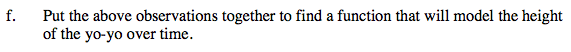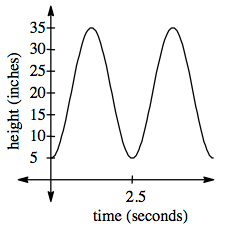### Home > PC > Chapter 6 > Lesson 6.1.1 > Problem6-11

6-11.
1. Jesse wants to model the height of a yo-yo over time by using a trigonometric function. He starts timing the yo-yo when it is at its lowest position of 5 inches above the ground. The string length is 30 inches and it took 2.5 seconds to return to the same position. Homework Help ✎

1.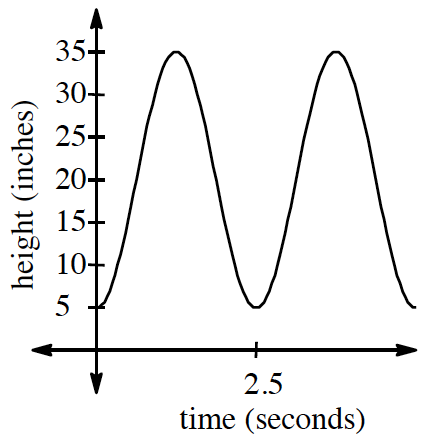The graph at right models the path of the yo-yo. Explain why it has a peak at 35 inches.

2. What is the amplitude of the function?

3. In order to find the vertical shift, we need to find the middle point between the high and low points. What is the vertical shift?

4. Recall the relationship pb = 2π. Find the angular frequency for the graph.

5. The graph can be modeled without utilizing a horizontal shift. Which trigonometric function can be used as a basis for this graph?

6. Put the above observations together to find a function that will model the height of the yo-yo over time.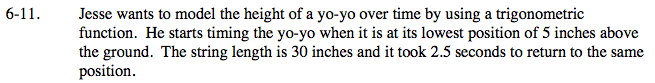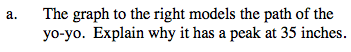Since the string is 30 inches in length, the maximum point will be 30 inches above the minimum.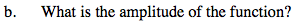Go from the middle of the curve to the highest point.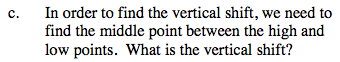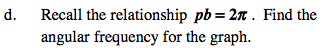The period is the x-distance of one cycle.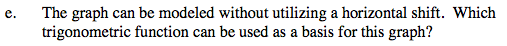−cos x